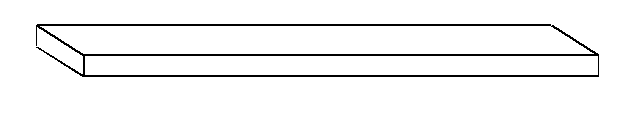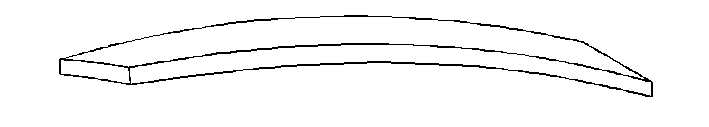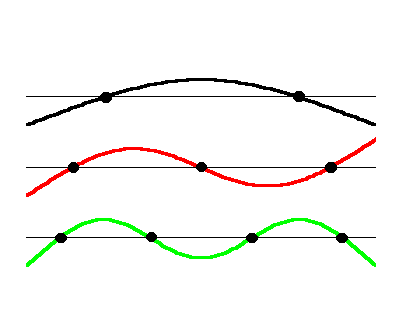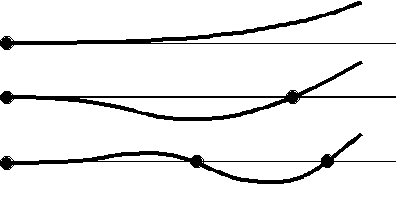4.1 Vibrating bars and Non-linear Dependencies

So far, we have only considered systems with harmonic overtones, the vibrating string and the air column. However, these are rather special, although important, cases. Many systems, including some musical instruments, do not have harmonic overtones.

Unlike strings, which vibrate because they are under tension, some instruments are based solely on stiffness, like the vibrating bars of a xylophone or a marimba. In this case, no tension needs to be applied to a bar for it to vibrate. For these instruments, the vibrational frequency depends on the stiffness of the bar, not the tension applied.

Yet another class of instruments depends on vibrations in 2 dimensions. This class includes many percussion instruments, like drums or cymbals. Here, the vibrating part of the instrument is a surface. We will return to this situation in a later section.

However, a general feature of vibrating bars and 2-dimensional instruments is that they are not so melodic and are generally used as percussion instruments. Why this is will be discussed in the next section.

Modes of vibration of a bar

What does a bar look like when it vibrates? The answer to this depends a lot on how the bar is held or supported. To start with, let’s just assume that we are on the space shuttle and the bar is floating freely in space:If it starts vibrating it will look something like this:The vibrational modes of a bar can get quite complex. It can bend in the long direction, the short direction or it can twist! Vibrating bars for instruments are made long and narrow so that the main bending is in the long direction. Twisting and the short direction are not so important. So, we will focus on just the one direction. In this case, it is easier to look at the bar from the side. Here are the first few modes of vibration:The exact shape of these modes cannot be determined without calculus, which is beyond the scope of this course, although, there are some features that we can recognize. There are still nodes and antinodes and there is one more node for each higher mode of vibration. However, the spacing between the nodes and antinodes is not the same. To get some sense of what the shape should be, just remember that the bar is very stiff and does not want to bend, so any part that is not forced to bend will be straight. Notice that the very ends of the bars always end up straight.

At this point, we usually write down the frequency of each mode relative to the fundamental. Again, there is no simple way to figure out the frequency of the higher modes without calculus, so we look up in a book and find that the overtones series is:

1f, 2.76f, 5.40f, 8.93f, …

This is certainly not a simple harmonic series. But, we will not take it for granted! We will test this out in one of the labs.

Just as with an air column, we can have different boundary conditions at the ends of the bars: the ends can be free to vibrate, or they can be clamped. If the end is clamped, there will be a node and if it is free there will be an antinode. And these can be combined in various ways, just like the air column: free-free, free-clamped, and clamped-clamped. This last one, clamped-clamped is highly damped and not that useful. However, the free-clamped combination has an interesting application. These are what its modes look like:The overtones series for this arrangement is: 1f, 5.3f, 12.7f, … Notice how much higher the first overtone is compared to the fundamental. Here, the shape of the modes is clearly much different from a vibrating string or air column. Actually, if you have ever tried to twirl a chain or heavy string around, you may notice that it takes on the same modes as the bar clamped at one end.

Dependence of frequency on length

Once we have the fundamental, we can predict the frequency of the higher modes. What does the fundamental depend on? Thickness, width, material, length, etc. The actually frequency of the fundamental of a vibrating bar is much harder to write down than for a vibrating string, so we will not do this. Rather, we will focus in on just one of the dependences: how does the fundamental change with the length of the bar.

Remember that for a string, if you double the length, the frequency goes down by a factor of two. So, the frequency, f, depends on length, L, in the following way: f = constant/L. We would say that the frequency in linearly but inversely proportional to the length. Also, remember, the string had harmonic overtones and the distance between nodes is always the same, in a particular mode.

As we have seen, the overtones of a bar are not harmonic and the modes were more complicated than for a string. So, it is reasonable to ask whether the fundamental of the bar will vary with length in the same way that the string does. In fact, the fundamental does not have the same dependence on length. All’s that we have to do is look at a toy (or real) xylophone!

If we look at a single octave of the xylophone, the frequency changes by a factor of two. But if we look at the lengths of the bars an octave apart, the upper note is not close to one half of the length of the lower note. It is longer than we would expect, close to three quarters of the length of the lower note. If we carefully measure the lengths of the bars, we would find that they are in a ratio of 0.707 to 1. So the shorter the bar the higher the frequency, just like a string, but where does the 0.707 come from? As it turns out, (0.707)2 = 0.5 = 1/2. This is the ratio of the frequencies! So, it turns out that the frequency depends on the SQUARE of the length. If you change the length by a factor of 0.707, the frequency changes by a factor of (0.707)2. How can we express this as a relationship? We can write:

f = constant/L2.

Here, the constant hides all the other complexities of determining the frequency of the bar. This relationship simply highlights the dependence on length. As an exercise, predict by what factor you must shorten the length of a bar to raise its pitch by a Perfect Fifth. You may have to review Section 2.3 to do this.

Real xylophones and vibraphones are yet more complicated. It was shown in a project for this class that the lengths of the bars did not exactly follow the relationship above. It turns out that instrument makers shape the bars by thinning them in the middle, to change the overtone series. This can make the bars sound a little better, but this also changes the pitch of the fundamental. So, the dependence on length does not hold perfectly for real instruments.